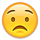## Forum Stats

• 3,734,034 Users
• 2,246,862 Discussions
• 7,857,003 Comments

Discussions

#### Howdy, Stranger!

It looks like you're new here. If you want to get involved, click one of these buttons!

# Sorting a Column

I have a table that i get this data out of

1
2
3
4
5
6

I Then break it up into 2 columns
1 4
2 5
3 6

What i would like to be able to do is have some way of making the 2nd column display
6
5
4

Is there some basic command that can do this to a column.

It cant be done by using order by in my case, as the inital column is ordered by and this would throw everything out of whack.

Any one know anything about this?

with t as
(SELECT (FamilyName || ' ' || GivenName) as nom, row_number() over (order by DateOfBirth ASC) as rn from member)
select t1.nom as leftside, t2.nom as rightside
from t t1, t t2
where rownum < (SELECT COUNT(memberNo) / 2 + 1 AS table_length FROM Member) and
t1.rn != t2.rn
and mod(t1.rn,(SELECT COUNT(memberNo) / 2 AS table_length FROM Member)) = mod(t2.rn, (SELECT COUNT(memberNo) / 2 AS table_length FROM Member));

this is the sql code that i currently have.

Message was edited by:
user636973

## Comments

• How about something like this?
```  1  with t as (
2     select rownum as rn1
3     , row_number() over (order by rownum desc) rn2
4     , trunc(count(*) over () / 2) as mid
5     from all_objects where rownum <= 6
6  )
7  select rn1 as leftside
8  , rn2 as rightside
9  from t
10  where rn1 <= mid
11* order by rn1
SQL> /
LEFTSIDE  RIGHTSIDE
---------- ----------
1          6
2          5
3          4```
cheers,
Anthony
• ```with t as ( select level*101 as x from dual connect by level <= 7),
tt as ( select x, row_number() over (order by x) - 1 as rn, ceil(count(*) over () / 2) as win from t)
select min(decode(trunc(rn/win), 0, x)) as x1,
min(decode(trunc(rn/win), 1, x)) as x2
from tt
group by mod(rn, win)
order by mod(rn, win)
;

X1            X2
------------- -------------
101           505
202           606
303           707
404```
• It seems to me as if you try to force a client issue (layout thingie) onto the database server.

The displaying of select results should be handled by the client. Most client tools have enough capabilities to do such a thing. It is generally a bad idea to do the whole layouting in the select already.

Split the result set and print the second part in reversed order.

btw: Same idea goes for stuff like pivot querys.
• Currently your MOD(t1.rn, ...) = MOD(t2.rn,...)

is what is giving you

1 4
2 5
3 6

because that's what you asked me for when I supplied this query in your previous thread.

Now MOD is the wrong approach. You want

1 COUNT
2 COUNT-1
3 COUNT-2

So replace the MOD comparison with

AND t2.rn(+) = (SELECT count(1) from member) - ( t1.rn - 1 )
ORDER BY t1.rn

The outer join is so that if there are an odd number of rows, t1 value will be present and t2 value will be null for the last row.

Message was edited by:
Dave Hemming

Using aketi's test table query would be
```with t as
(SELECT val as nom, row_number() over (order by val ASC) as rn from testT)
select t1.nom as leftside, decode(t2.nom,t1.nom,null,t2.nom) as rightside
from t t1, t t2
where rownum < (SELECT COUNT(val) / 2 + 1 AS table_length FROM testt)
AND t2.rn(+) = (SELECT count(1) from testt) - ( t1.rn - 1 )```
• This is an interesting question.
```create table testT(Val) as
select RowNum*10 from all_catalog
where RowNum <=7;```
```select Left,Right
from (select Rn,Cnt,Val as Left,
case when Rn <= Cnt/2
then Lead(Val,abs(Cnt-2*Rn+1)) over(order by Val) end as Right
from (select Val,
Row_Number() over(order by Val) as Rn,
count(*) over() as Cnt
from testT))
where Rn <= ceil(Cnt/2);```
```LEFT  RIGHT
----  -----
10     70
20     60
30     50
40   null```
• OopsMisread
```with t as ( select level*101 as x from dual connect by level <= 7),
tt as ( select x, row_number() over (order by x) - 1 as rn, (count(*) over () - 1) / 2 as median_rn from t)
select min(case when rn <= median_rn then x end) as x1,
min(case when rn >  median_rn then x end) as x2
from tt
group by abs(rn - median_rn)
order by abs(rn - median_rn) desc
;

X1            X2
------------- -------------
101           707
202           606
303           505
404```
• `>   group by abs(rn - median_rn)`
Another variation of odd count:
```  group by abs(trunc(median_rn - rn + 0.1))

X1            X2
------------- -------------
101
202           707
303           606
404           505```
This discussion has been closed.